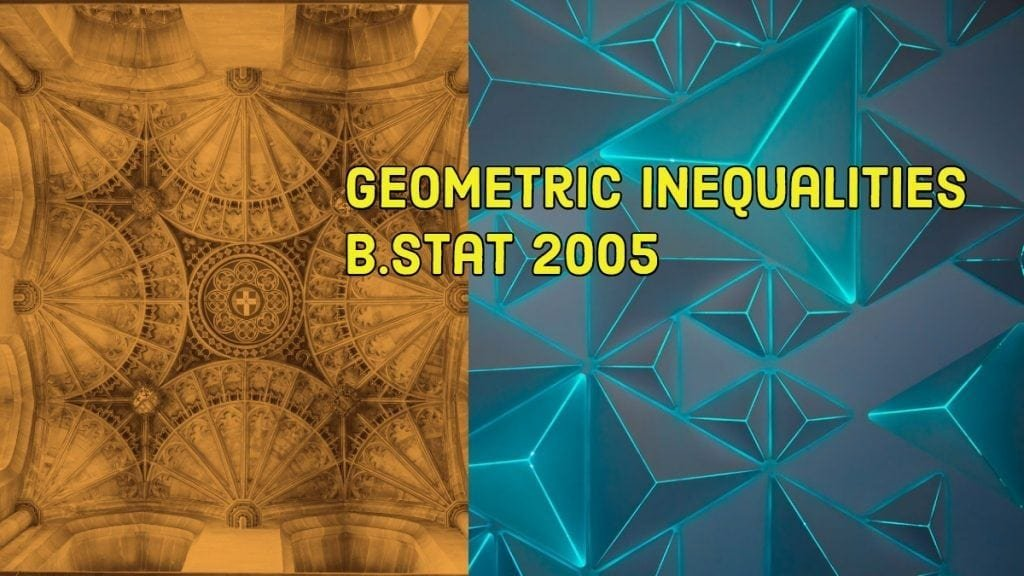How Cheenta works to ensure student success?
Explore the Back-Story

# Geometric inequality | I.S.I. B.Stat 2005 Problem 5 solutionThis is problem number 5 from Indian Statistical Institute, ISI BStat 2005 based on Geometric inequality.

Consider an acute angled triangle $PQR$ such that $C,I$ and $O$ are the circumcentre, incentre and orthocentre respectively. Suppose $\displaystyle{ \angle QCR, \angle QIR }$ and $\displaystyle{ \angle QOR }$, measured in degrees, are $\displaystyle{ \alpha, \beta }$ and $\gamma$ respectively. Show that $\displaystyle { \frac{1}{\alpha}+\frac{1}{\beta}+\frac{1}{\gamma} > \frac{1}{45} }$

Discussion:

Teacher:

Use this diagram. Angle chasing should suffice. Assume the that the measure of $\angle PQR = Q , \angle RPQ = P , \angle PRQ = R$

Student: $\alpha = 2P$ since it is the angle at the center (twice the angle at circumference). Again $\displaystyle { \angle IQR = \frac{Q}{2} , \angle IRQ = \frac {R}{2} \Rightarrow \beta = 180^{\circ} - \frac{Q+R}{2} = 90^{\circ} + \frac{P}{2} }$
Finally $\angle BQR = \angle OQR = 90^{\circ} -R, \angle ORQ = 90^{\circ} - Q \Rightarrow \gamma = R+Q$

Hence $\displaystyle { \frac{1}{\alpha}+\frac{1}{\beta}+\frac{1}{\gamma} = \frac{1}{2P}+\frac{1}{90} + \frac{P}{2}+\frac{1}{180-P} }$

But here is a problem. If $I$ maximize $P$ (by replacing it $90$), it adversely affects $180 - P$ as $P$ is negative there. Somehow we must balance the value of $P$.

Teacher: Right. If you replace $P$ by $90$, $\frac{1}{2P}$ will remain greater than $\frac{1}{180}$, but $\frac{1}{90}$ will be less than $\frac{1}{180}-P$.
Here we need to use Arithmetic Mean > Harmonic Mean inequality which state for positive numbers $a, b, c$ we have $\displaystyle { \frac{3}{\frac{1}{a}+ \frac{1}{b} + \frac{1}{c} } \leq \frac{a+b+c}{3} \Rightarrow \frac{9}{a+b+c} \leq \frac{1}{a}+ \frac{1}{b} + \frac{1}{c} }$

$\displaystyle { \frac{1}{\alpha}+\frac{1}{\beta}+\frac{1}{\gamma} = \frac{1}{2P}+\frac{1}{90} + \frac{P}{2}}+\frac{1}{180-P} \geq \frac{9} { 2P +90 + \frac{P}{2} + 180 - P }$

Student: Ok. I think I can do it from here.

Since it is an acute angled triangle, $P \leq 90^{\circ}$
Hence replacing $P$ by $90$, we get

$\displaystyle { \frac{1}{\alpha}+\frac{1}{\beta}+\frac{1}{\gamma} > \frac{9} { 90 +90 + \frac{90}{2} + 180 } = \frac{9}{9 \times 45} = \frac{1}{45} }$

Teacher: Just one point of caution; all numbers are in degrees. Also mention that 'increasing the denominator decreases a number'.

This is problem number 5 from Indian Statistical Institute, ISI BStat 2005 based on Geometric inequality.

Consider an acute angled triangle $PQR$ such that $C,I$ and $O$ are the circumcentre, incentre and orthocentre respectively. Suppose $\displaystyle{ \angle QCR, \angle QIR }$ and $\displaystyle{ \angle QOR }$, measured in degrees, are $\displaystyle{ \alpha, \beta }$ and $\gamma$ respectively. Show that $\displaystyle { \frac{1}{\alpha}+\frac{1}{\beta}+\frac{1}{\gamma} > \frac{1}{45} }$

Discussion:

Teacher:

Use this diagram. Angle chasing should suffice. Assume the that the measure of $\angle PQR = Q , \angle RPQ = P , \angle PRQ = R$

Student: $\alpha = 2P$ since it is the angle at the center (twice the angle at circumference). Again $\displaystyle { \angle IQR = \frac{Q}{2} , \angle IRQ = \frac {R}{2} \Rightarrow \beta = 180^{\circ} - \frac{Q+R}{2} = 90^{\circ} + \frac{P}{2} }$
Finally $\angle BQR = \angle OQR = 90^{\circ} -R, \angle ORQ = 90^{\circ} - Q \Rightarrow \gamma = R+Q$

Hence $\displaystyle { \frac{1}{\alpha}+\frac{1}{\beta}+\frac{1}{\gamma} = \frac{1}{2P}+\frac{1}{90} + \frac{P}{2}+\frac{1}{180-P} }$

But here is a problem. If $I$ maximize $P$ (by replacing it $90$), it adversely affects $180 - P$ as $P$ is negative there. Somehow we must balance the value of $P$.

Teacher: Right. If you replace $P$ by $90$, $\frac{1}{2P}$ will remain greater than $\frac{1}{180}$, but $\frac{1}{90}$ will be less than $\frac{1}{180}-P$.
Here we need to use Arithmetic Mean > Harmonic Mean inequality which state for positive numbers $a, b, c$ we have $\displaystyle { \frac{3}{\frac{1}{a}+ \frac{1}{b} + \frac{1}{c} } \leq \frac{a+b+c}{3} \Rightarrow \frac{9}{a+b+c} \leq \frac{1}{a}+ \frac{1}{b} + \frac{1}{c} }$

$\displaystyle { \frac{1}{\alpha}+\frac{1}{\beta}+\frac{1}{\gamma} = \frac{1}{2P}+\frac{1}{90} + \frac{P}{2}}+\frac{1}{180-P} \geq \frac{9} { 2P +90 + \frac{P}{2} + 180 - P }$

Student: Ok. I think I can do it from here.

Since it is an acute angled triangle, $P \leq 90^{\circ}$
Hence replacing $P$ by $90$, we get

$\displaystyle { \frac{1}{\alpha}+\frac{1}{\beta}+\frac{1}{\gamma} > \frac{9} { 90 +90 + \frac{90}{2} + 180 } = \frac{9}{9 \times 45} = \frac{1}{45} }$

Teacher: Just one point of caution; all numbers are in degrees. Also mention that 'increasing the denominator decreases a number'.

This site uses Akismet to reduce spam. Learn how your comment data is processed.

### One comment on “Geometric inequality | I.S.I. B.Stat 2005 Problem 5 solution”

1. […] Geometric inequality (I.S.I. B.Stat 2005 Problem 5 solution) […]

### Knowledge Partner